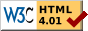## Dokumentation zu: clear_bit(E)``` SYNOPSIS string clear_bit(string str, int n) DESCRIPTION Return the new string where bit n is cleared in string str. Note that the old string str is not modified. Each character contains 6 bits. So you can store a value between 0 and 63 ( 2^6=64) in one character. Starting character is the blank character " " which has the value 0. The first charcter in the string is the one with the lowest bits (0-5). EXAMPLES string s; s=clear_bit("_",5); Because "_" is the highest possible value (63), the variable s will now contain the charcter "?" wich is equal to 31 (63-2^5=31). string s; s=clear_bit("?<",3); s=clear_bit(s,8); s will now contain the string "78". "?" equals 31 and "<" equals 28. Now "?<" is equal to 31+28<<6=31+1792=1823 which is in binary notation (highest bit on the right side) 11111000111. Now clearing the bit 3 and bit 8 (bit numbering starts with zero) will result in 11101000011. The first 6 bits are in decimal notation 23 and the next 6 are equal to 24. Now the 23 is the character "7" and 24 is the "8". So the string s contains "78". SEE ALSO set_bit(E), next_bit(E), last_bit(E), test_bit(E), count_bits(E), and_bits(E), or_bits(E), xor_bits(E), invert_bits(E), copy_bits(E) ``` Die Seite ist auch in Deutsch vorhanden.

Start » Magierhandbuch » Docu » Efun » Clear_bit Letzte Generierung: 25.04.2021, 01:58mud@wl.mud.de